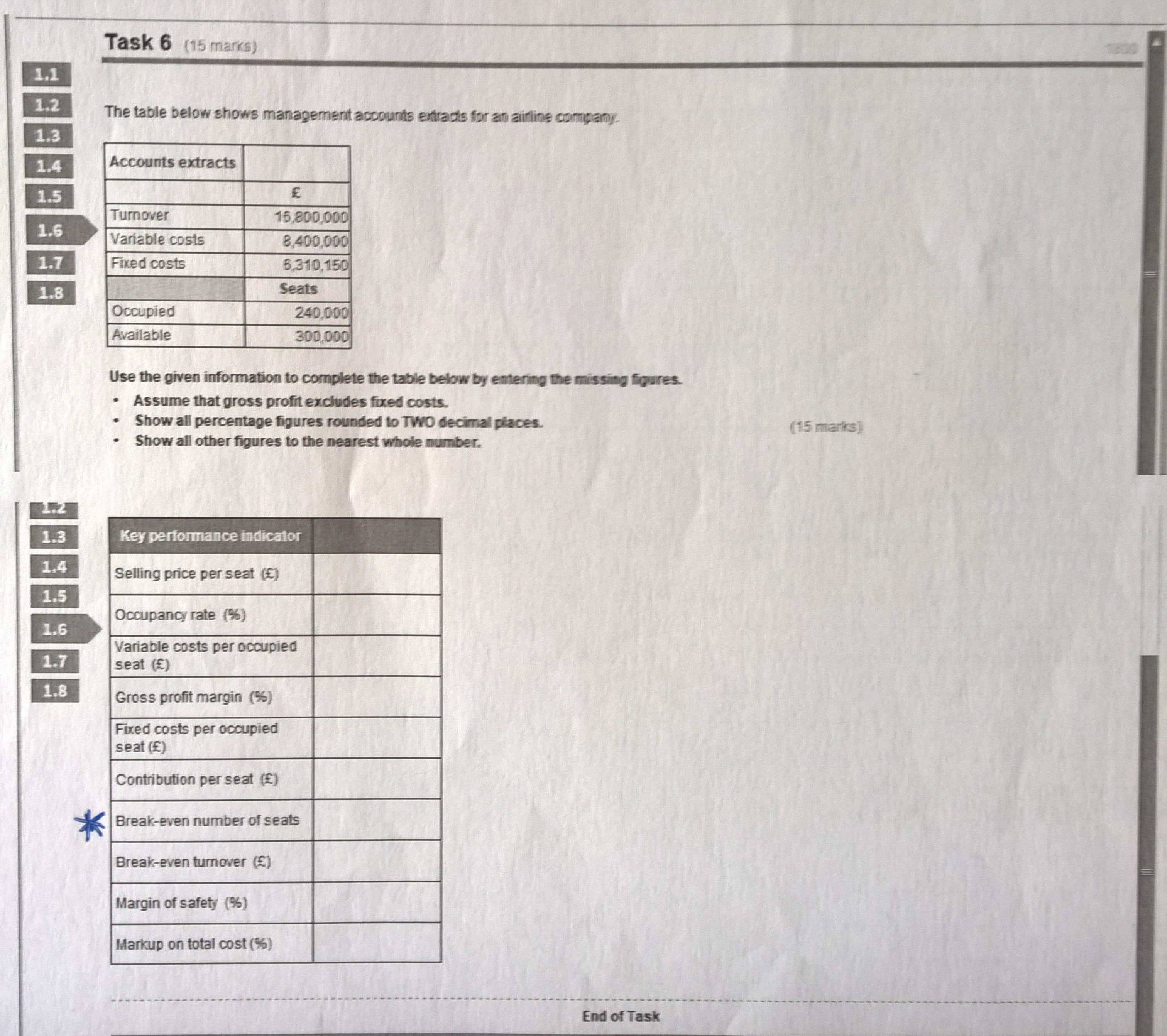# Practice Assessment 2 - Task 6

Hi,

Any help would be much appreciated! Thank you!• @Zana I am assuming you mean the starred question ? Breakeven = Fixed costs/ contribution. so in this case 6310150/35(contribution per seat)= 180290.

To get the 35 ( selling price per seat-contribution per seat) so £70-£35

Hope this helps• Hi @Lyndsey1570, thank you! do you think you could help me with the rest as well? The star was there just for me-question, that I am struggling with... I am sorry for the confusion...
• no problem @Zana sorry here's the breakdown of how i worked this answer outSo Selling price per seat = Turnover/occupied seats (16800000/240000) = £70 per seat
Occupancy rate %= occupied seats/ available x 100 (240,000/300,000 x 100) = 80%
Variable cost per seat occupied= variable costs/ occupied seats (8400000/240000) = £35
Gross profit margin (says in question assume GP excludes fixed costs)= Variable costs/turnover (8400000/16800000 x 100) = 50%
Fixed costs per seat occupied = fixed costs/ occupied seats = (6310150/240000)= £26 remember to round up to nearest whole number
Contribution per seat = Sales price-variable costs = £70-£35=£35
Breakeven = see above answerBreakeven turnover £ breakeven in seats x sales price = 180290 x £70= £12620300
Margin of safety = Occupied seats - breakeven/ occupied seats x 100 so 240,000- 180290/240000 x 100 = 24.88%
Mark up on total cost = profit/total cost x 100 = turnover - variable costs- fixed costs = (16800000-8400000-6310150) = 2089850
Total costs = variable costs + fixed costs = 14710150
Therefore answer is 2089850/1470150 x 100 = 14.21%

Any questions just let me know sorry if i have laid this out really confusing Goodluck for the exam whenever it is!
• Wonderful! Thanks a lot @lyndsey1570 !!!